Processing ......FreeComputerBooks.com Links to Free Computer, Mathematics, Technical Books all over the World

Optimization Algorithms on Matrix Manifolds
Top Free Python Books 🌠 - 100% Free or Open Source!
• Title Optimization Algorithms on Matrix Manifolds
• Authors P.-A. Absil, R. Mahony, R. Sepulchre
• Publisher: Princeton University Press; illustrated edition edition (December 3, 2008)
• Paperback: 240 pages
• Language: English
• ISBN-10: 0691132984
• ISBN-13: 978-0691132983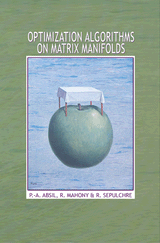Book Description

Many problems in the sciences and engineering can be rephrased as optimization problems on matrix search spaces endowed with a so-called manifold structure. This book shows how to exploit the special structure of such problems to develop efficient numerical algorithms. It places careful emphasis on both the numerical formulation of the algorithm and its differential geometric abstraction--illustrating how good algorithms draw equally from the insights of differential geometry, optimization, and numerical analysis. Two more theoretical chapters provide readers with the background in differential geometry necessary to algorithmic development. In the other chapters, several well-known optimization methods such as steepest descent and conjugate gradients are generalized to abstract manifolds. The book provides a generic development of each of these methods, building upon the material of the geometric chapters. It then guides readers through the calculations that turn these geometrically formulated methods into concrete numerical algorithms. The state-of-the-art algorithms given as examples are competitive with the best existing algorithms for a selection of eigenspace problems in numerical linear algebra.

Optimization Algorithms on Matrix Manifolds offers techniques with broad applications in linear algebra, signal processing, data mining, computer vision, and statistical analysis. It can serve as a graduate-level textbook and will be of interest to applied mathematicians, engineers, and computer scientists.

The treatment strikes an appropriate balance between mathematical, numerical, and algorithmic points of view. The quality of the writing is quite high and very readable. The topic is very timely and is certainly of interest to myself and my students."--Kyle A. Gallivan, Florida State University

• P.-A. Absil is associate professor of mathematical engineering at the Universite Catholique de Louvain in Belgium. R. Mahony is reader in engineering at the Australian National University. R. Sepulchre is professor of electrical engineering and computer science at the University of Liege in Belgium.
Reviews and Rating: Related Book Categories: Read and Download Links:Similar Books:
•Manifolds - Current Research Areas (Paul Bracken)

This book cover a number of subjects which will be of interest to workers in these areas. It is hoped that the papers here will be able to provide a useful resource for researchers with regard to current fields of research in this important area.

•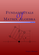Fundamentals of Matrix Algebra (Gregory Hartman)

A college (or advanced high school) level text dealing with the basic principles of matrix and linear algebra. It covers solving systems of linear equations, matrix arithmetic, the determinant, eigenvalues, and linear transformations.

•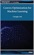Convex Optimization for Machine Learning (Changho Suh)

This book covers an introduction to convex optimization, one of the powerful and tractable optimization problems that can be efficiently solved on a computer. The goal is to help develop a sense of what convex optimization is, and how it can be used.

•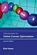Introduction to Online Convex Optimization (Elad Hazan)

This book presents a robust machine learning approach that contains elements of mathematical optimization, game theory, and learning theory: an optimization method that learns from experience as more aspects of the problem are observed.

•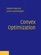Convex Optimization (Stephen Boyd, et al.)

On recognizing convex optimization problems and then finding the most appropriate technique for solving them. It contains many worked examples in fields such as engineering, computer science, mathematics, statistics, finance, and economics.

•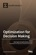Optimization for Decision Making: Linear and Quadratic Models

This book illustrates how to formulate real world problems using linear and quadratic models; It focus on developing modeling skills to support valid decision making for complex real world problems.

•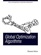Global Optimization Algorithms - Theory and Application

This book is devoted to global optimization algorithms, which are methods to find optimal solutions for given problems. It especially focuses on Evolutionary Computation by discussing evolutionary algorithms, genetic algorithms, Genetic Programming, etc.

•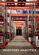Inventory Analytics: A Practicable, Python-Driven Approach

This book provides a comprehensive and accessible introduction to the theory and practice of Inventory Control - a significant research area central to supply chain planning. It adopts a practicable, Python-driven approach to illustrating theories and concepts.

•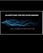Algorithms for Decision Making (Mykel Kochenderfer, et al)

This textbook provides a broad introduction to algorithms for decision making under uncertainty, covering the underlying mathematical problem formulations and the algorithms for solving them.

Book Categories
 :All CategoriesTop Free BooksRecent BooksMiscellaneous BooksComputer EngineeringComputer LanguagesComputer ScienceData Science/DatabasesJava and Java EE (J2EE)Linux and UnixMathematicsMicrosoft and .NETMobile ComputingNetworking and CommunicationsSoftware EngineeringSpecial TopicsWeb Programming
Other Categories